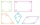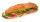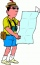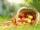# Fraction calculator

The calculator performs basic and advanced operations with fractions, expressions with fractions combined with integers, decimals, and mixed numbers. It also shows detailed step-by-step information about the fraction calculation procedure. Solve problems with two, three, or more fractions and numbers in one expression.

## Result:

### 7 * 5/6 = 35/6 = 5 5/6 ≅ 5.8333333

Spelled result in words is thirty-five sixths (or five and five sixths).

### How do you solve fractions step by step?

1. Multiple: 7 * 5/6 = 7 · 5/1 · 6 = 35/6
Multiply both numerators and denominators. Result fraction keep to lowest possible denominator GCD(35, 6) = 1. In the next intermediate step, the fraction result cannot be further simplified by canceling.
In words - seven multiplied by five sixths = thirty-five sixths.

#### Rules for expressions with fractions:

Fractions - use the slash “/” between the numerator and denominator, i.e., for five-hundredths, enter 5/100. If you are using mixed numbers, be sure to leave a single space between the whole and fraction part.
The slash separates the numerator (number above a fraction line) and denominator (number below).

Mixed numerals (mixed fractions or mixed numbers) write as non-zero integer separated by one space and fraction i.e., 1 2/3 (having the same sign). An example of a negative mixed fraction: -5 1/2.
Because slash is both signs for fraction line and division, we recommended use colon (:) as the operator of division fractions i.e., 1/2 : 3.

Decimals (decimal numbers) enter with a decimal point . and they are automatically converted to fractions - i.e. 1.45.

The colon : and slash / is the symbol of division. Can be used to divide mixed numbers 1 2/3 : 4 3/8 or can be used for write complex fractions i.e. 1/2 : 1/3.
An asterisk * or × is the symbol for multiplication.
Plus + is addition, minus sign - is subtraction and ()[] is mathematical parentheses.
The exponentiation/power symbol is ^ - for example: (7/8-4/5)^2 = (7/8-4/5)2

#### Examples:

subtracting fractions: 2/3 - 1/2
multiplying fractions: 7/8 * 3/9
dividing Fractions: 1/2 : 3/4
exponentiation of fraction: 3/5^3
fractional exponents: 16 ^ 1/2
adding fractions and mixed numbers: 8/5 + 6 2/7
dividing integer and fraction: 5 ÷ 1/2
complex fractions: 5/8 : 2 2/3
decimal to fraction: 0.625
Fraction to Decimal: 1/4
Fraction to Percent: 1/8 %
comparing fractions: 1/4 2/3
multiplying a fraction by a whole number: 6 * 3/4
square root of a fraction: sqrt(1/16)
reducing or simplifying the fraction (simplification) - dividing the numerator and denominator of a fraction by the same non-zero number - equivalent fraction: 4/22
expression with brackets: 1/3 * (1/2 - 3 3/8)
compound fraction: 3/4 of 5/7
fractions multiple: 2/3 of 3/5
divide to find the quotient: 3/5 ÷ 2/3

The calculator follows well-known rules for order of operations. The most common mnemonics for remembering this order of operations are:
PEMDAS - Parentheses, Exponents, Multiplication, Division, Addition, Subtraction.
BEDMAS - Brackets, Exponents, Division, Multiplication, Addition, Subtraction
BODMAS - Brackets, Of or Order, Division, Multiplication, Addition, Subtraction.
GEMDAS - Grouping Symbols - brackets (){}, Exponents, Multiplication, Division, Addition, Subtraction.
Be careful, always do multiplication and division before addition and subtraction. Some operators (+ and -) and (* and /) has the same priority and then must evaluate from left to right.

## Fractions in word problems:

• Sum of fractionsWhat is the sum of 2/3+3/5?
• DividendsThe three friends divided the win by the invested money. Karlos got three-eighths, John 320 permille, and the rest got Martin. Who got the most and which the least?
• Brick weightHalf the weight of a brick plus 20 pounds is equal to 1/3 the weight of the brick plus 30 pounds. How much does the brick weigh?
• PlayingHow long have we trained on the pitch when we know that the warm-up took 10 minutes, we trained passes for one-third of the time and we played football half the time?
• Series and sequencesFind a fraction equivalent to the recurring decimal? 0.435643564356
• PC disksPeter has 45 disks in three colors. One-fifth of the disks are blue, red are twice more than the white. How much is blue, red and white disks?
• The perimeter 2The perimeter of the quadrilateral a = 1m b = 14/5m c = 2 3/10m d = 1 4/5m?
• PocketMirka spent on a trip for gifts half pocket. For the third remain money bought a bagel. Six euros left her. How many euros had Mirka in pocket?
• WalkingLucie can walk about 3 4/5 miles each hour. How far can she walk in 2 hours 45 minutes?
• Dried applesHow many kilograms of fresh apples id needed to 120 kg of dried apples when from 0.4 tons of fresh apples we get 75 kg of dried apples?
• Equilateral triangle ABCIn the equilateral triangle ABC, K is the center of the AB side, the L point lies on one-third of the BC side near the point C, and the point M lies in the one-third of the side of the AC side closer to the point A. Find what part of the ABC triangle cont
• A class IV.AIn a class there are 15 girls and 30 boys.  What fraction of the class represents the boys?
• Barbara 2Barbara get 6 pizzas to divide equally among 4 people. How much of a pizza can each person have?